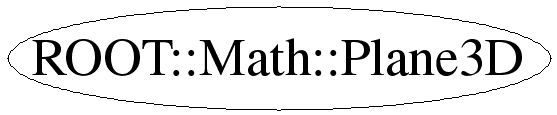# class ROOT::Math::Plane3D


Class describing a geometrical plane in 3 dimensions.
A Plane3D is a 2 dimensional surface spanned by two linearly independent vectors.
The plane is described by the equation
\f$a*x + b*y + c*z + d = 0 \f$ where (a,b,c) are the components of the
normal vector to the plane \f$n = (a,b,c) \f$ and \f$d = - n \dot x \f$, where x is any point
belonging to plane.
More information on the mathematics describing a plane in 3D is available on
<A HREF=http://mathworld.wolfram.com/Plane.html>MathWord</A>.
The Plane3D class contains the 4 scalar values in double which represent the
four coefficients, fA, fB, fC, fD. fA, fB, fC are the normal components normalized to 1,
i.e. fA**2 + fB**2 + fC**2 = 1

@ingroup GenVector



## Function Members (Methods)

public:
 ~Plane3D() ROOT::Math::Plane3D::Scalar A() ROOT::Math::Plane3D::Scalar B() ROOT::Math::Plane3D::Scalar C() ROOT::Math::Plane3D::Scalar D() ROOT::Math::Plane3D::Scalar Distance(const ROOT::Math::Plane3D::Point& p) const ROOT::Math::Plane3D::Scalar HesseDistance() const ROOT::Math::Plane3D::Vector Normal() const bool operator!=(const ROOT::Math::Plane3D& rhs) const ROOT::Math::Plane3D& operator=(const ROOT::Math::Plane3D& plane) bool operator==(const ROOT::Math::Plane3D& rhs) const ROOT::Math::Plane3D Plane3D() ROOT::Math::Plane3D Plane3D(const ROOT::Math::Plane3D&) ROOT::Math::Plane3D Plane3D(const ROOT::Math::Plane3D::Vector& n, const ROOT::Math::Plane3D::Point& p) ROOT::Math::Plane3D Plane3D(const ROOT::Math::Plane3D::Point& p1, const ROOT::Math::Plane3D::Point& p2, const ROOT::Math::Plane3D::Point& p3) ROOT::Math::Plane3D Plane3D(const ROOT::Math::Plane3D::Scalar& a, const ROOT::Math::Plane3D::Scalar& b, const ROOT::Math::Plane3D::Scalar& c, const ROOT::Math::Plane3D::Scalar& d) ROOT::Math::Plane3D::Point ProjectOntoPlane(const ROOT::Math::Plane3D::Point& p) const
protected:
 void Normalize()

## Data Members

private:
 ROOT::Math::Plane3D::Scalar fA ROOT::Math::Plane3D::Scalar fB ROOT::Math::Plane3D::Scalar fC ROOT::Math::Plane3D::Scalar fD

## Class Charts## Function documentation

D( )
default constructor create plane z = 0


{ }
Plane3D(const ROOT::Math::Plane3D::Scalar& a, const ROOT::Math::Plane3D::Scalar& b, const ROOT::Math::Plane3D::Scalar& c, const ROOT::Math::Plane3D::Scalar& d)
generic constructors from the four scalar values describing the plane
according to the equation ax + by + cz + d = 0
\param a scalar value
\param b scalar value
\param c scalar value
\param d sxcalar value


Plane3D(const ROOT::Math::Plane3D::Vector& n, const ROOT::Math::Plane3D::Point& p)
constructor a Plane3D from a normal vector and a point coplanar to the plane
\param n normal expressed as a ROOT::Math::DisplacementVector3D<Cartesian3D<double> >
\param p point  expressed as a  ROOT::Math::PositionVector3D<Cartesian3D<double> >


Plane3D(const ROOT::Math::Plane3D::Scalar& a, const ROOT::Math::Plane3D::Scalar& b, const ROOT::Math::Plane3D::Scalar& c, const ROOT::Math::Plane3D::Scalar& d)
Construct from a generic DisplacementVector3D (normal vector) and PositionVector3D (point coplanar to
the plane)
\param n normal expressed as a generic ROOT::Math::DisplacementVector3D
\param p point  expressed as a generic ROOT::Math::PositionVector3D


{}
Plane3D(const ROOT::Math::Plane3D::Point& p1, const ROOT::Math::Plane3D::Point& p2, const ROOT::Math::Plane3D::Point& p3)
constructor from three Cartesian point belonging to the plane
\param p1 point1  expressed as a generic ROOT::Math::PositionVector3D
\param p2 point2  expressed as a generic ROOT::Math::PositionVector3D
\param p3 point3  expressed as a generic ROOT::Math::PositionVector3D


Plane3D(const PositionVector3D<T1,U> & p1, const PositionVector3D<T2,U> & p2, const PositionVector3D<T3,U> & p3 )
constructor from three generic point belonging to the plane
\param p1 point1 expressed as  ROOT::Math::DisplacementVector3D<Cartesian3D<double> >
\param p2 point2 expressed as  ROOT::Math::DisplacementVector3D<Cartesian3D<double> >
\param p3 point3 expressed as  ROOT::Math::DisplacementVector3D<Cartesian3D<double> >


{}
Plane3D & operator=(const ROOT::Math::Plane3D& plane)
 compiler-generated copy ctor and dtor are fine.
------ assignment ------

Assignment operator from other Plane3D class


Scalar A()
Return the a coefficient of the plane equation \f$a*x + b*y + c*z + d = 0 \f$. It is also the
x-component of the vector perpendicular to the plane.


{ return fA; }
Scalar B()
Return the b coefficient of the plane equation \f$a*x + b*y + c*z + d = 0 \f$. It is also the
y-component of the vector perpendicular to the plane


{ return fB; }
Scalar C()
Return the c coefficient of the plane equation \f$a*x + b*y + c*z + d = 0 \f$. It is also the
z-component of the vector perpendicular to the plane


{ return fC; }
Vector Normal()
Return normal vector to the plane as Cartesian DisplacementVector


Scalar HesseDistance()
Return the Hesse Distance (distance from the origin) of the plane or
the d coefficient expressed in normalize form


Scalar Distance(const ROOT::Math::Plane3D::Point& p) const
Return the signed distance to a Point.
The distance is signed positive if the Point is in the same side of the
normal vector to the plane.
\param p Point expressed in Cartesian Coordinates


Point ProjectOntoPlane(const ROOT::Math::Plane3D::Point& p) const
Return the projection of a Cartesian point to a plane
\param p Point expressed as PositionVector3D<Cartesian3D<double> >


bool operator==(const ROOT::Math::Plane3D& rhs) const
 ------------------- Equality -----------------

Exact equality


bool operator!=(const ROOT::Math::Plane3D& rhs) const
void Normalize()
Normalize the normal (a,b,c) plane components



Last update: root/mathcore:$Id: Plane3D.h 21503 2007-12-19 17:34:54Z moneta$
Copyright (c) 2005 , LCG ROOT MathLib Team *

This page has been automatically generated. If you have any comments or suggestions about the page layout send a mail to ROOT support, or contact the developers with any questions or problems regarding ROOT.